# Double Stratification in the Flow of a Newtonian Fluid Along an Inclined Permeable Stretching Surface

Double Stratification in the Flow of a Newtonian Fluid Along an Inclined Permeable Stretching Surface
Department of Mathematics, National Institute of Technology, Warangal 506004, India

Corresponding Author Email:
chramreddy@nitw.ac.in
Page:
1-5
|
DOI:
https://doi.org/10.18280/ama_a.581-401
10 April 2021
|
Accepted:
12 August 2021
|
Published:
31 December 2021
| Citation

OPEN ACCESS

Abstract:

The impact of suction/injection on the doubly stratified Newtonian fluid flow along an inclined stretching surface through a porous medium with heat and mass flux conditions is investigated. Also, linear Boussinesq approximation is considered in this work to relate the transport phenomena of temperature and concentration in various thermal and solutal systems operated at moderate temperatures. The system of governing non-linear equations is changed to its dimensionless form using suitable transformation variables. The dimensionless non-linear ordinary differential equation system is linearized by local linearization approach and then the obtained linear equation system is dealt with Chebyshev collocation method. The illustrating analyses for the impact of the various physical parameters involved in the present study are provided and salient features are also discussed. The comparative studies of dimensionless skin friction coefficient, Nusselt number and Sherwood number are given with various set of values of various important parameters.

Keywords:

doubly stratified Newtonian fluid, suction/injection, stretching surface, porous medium, spectral method

1. Introduction

The Newtonian fluids are defined as the fluids in which at each point, there is a linear correlation between the viscous stress appearing due to its flow and the rate of change of deformation over time. This type of fluid is considered as one of the elementary mathematical models of fluids which takes care of viscosity. There are no such real fluids which suit perfectly to the definition, but several gases and liquids e.g., air and water, are presumed to show Newtonian behaviour in most of the realistic computations with simple conditions. In daily life, many examples of Newtonian fluid are found in the span of shear stresses with its rate, for example, glycerol, alcohol and thin motor oil etc. For more details, one can refer the book by Batchelor, C.K. and Batchelor, G.K. . A porous medium can be defined as a solid in which voids are scattered entirely in the bulk of the body and this scattering can be more or less uniform. Porosity is treated as the most basic materialistic property of porous medium and described as the ratio of void volume to the volume of solid. Permeability is the virtue which regulates the quantity of fluid flow across porous medium exposed to a pressure difference.

The studies related to heat and mass transfer problems involved in different porous media have been highly praised since last a few decades because of its various important applications in many industrial processes and in the field of engineering, for example, proper regulation of pile of nuclear waste, recycling of pollutant coming out of industry, food processing, etc. The books by Ingham and Pop  and Nield and Bejan  consist of detailed discussion about fluid flows along with heat and mass transfer. The studies related to different types of flows for various fluids over stretching sheet are found in literature from many decades ago. Crane  has discussed the flow over a stretching sheet and calculated the heat conduction in linear stretching case along with skin friction and heat transfer coefficients. Pal and Hiremath  considered an unsteady stretching surface and performed computational modelling of heat transfer. Also, in a nanofluid saturated non-Darcy porous medium, Soret effect on stagnation-point flow over a stretching/shrinking surface is discussed by RamReddy et al. . The different analyses related to boundary layer flow over an inclined stretching sheet have acquired considerable importance in last a few years due to its involvement in many important applications for various sectors of industries and engineering, e.g., as in production of papers, glass-fibre, drawing of wire and plastic films, in order to cool the metallic sheets using cool bath, etc. To get some more insights, one can refer the literature [7, 8] where various effects and their importance are given for flows over inclined stretching surfaces.

There is a certain type of fluid flow in which density of the fluid depends upon the gravity and so the fluid with higher density is always below the fluid with lower density. This type of fluid flow is called as stratified flow. The influences of double stratification on flows over various geometries for different fluids are studied by many researchers in recent years. Double diffusive non-Darcian free convection to porous medium from a wavy vertical wall is considered by Rathish Kumar  and they discussed the collective impact of thermal and solutal stratification on this flow in detail. The study of heat and mass transfer in free convection with double stratification is discussed by Lakshmi Narayana and Murthy . The impact of double stratification in mixed convective flow over a vertical plate is presented by Srinivasacharya and Surender . The impact of double stratification in the presence of Joule heating and thermal radiation for a flow caused by curved stretching sheet is presented by Hayat et al. .

Main objective in this article is to get idea about the impact of double stratification on laminar Newtonian fluid flow along an inclined stretching surface immersed in a porous medium with heat and mass flux conditions. The final non-dimensional non-linear ODEs obtained are solved with spectral local linearization method (SLLM) and effect of physical parameters is discussed with the aid of plots.

2. Formulation of the Problem

The two-dimensional, laminar and steady flow of incompressible and viscous fluid over an inclined stretching porous surface making an acute angle A immersed in a porous medium, is considered. The moving velocity of this porous surface is taken as U=ax (here a is fixed and termed as stretching rate). Suppose x-axis is the direction of inclined stretching sheet’s leading edge and y-axis is in its perpendicular direction. The linear stratification of temperature and concentration are given by $T_{\infty}(x)=T_{\infty, 0}+A^{\prime} x, C_{\infty}(x)=C_{\infty, 0}+B^{\prime} x$, where $A^{\prime}$ and $B^{\prime}$ are stratification intensity parameter. The Boussinesq’s approximation is also incorporated in the problem formulation. Gray and Giorgini  have given a detailed description about the Boussinesq’s approximation and its validity for liquids and gases.

Under all these assumptions and approximations, the governing equations are:

$\frac{\partial u}{\partial x}+\frac{\partial v}{\partial y}=0$     (1)

\begin{align} & \frac{1}{{{\varepsilon }^{2}}}\left( u\frac{\partial u}{\partial x}+v\frac{\partial v}{\partial y} \right)=\frac{v}{\varepsilon }\frac{{{\partial }^{2}}u}{\partial {{y}^{2}}}-\frac{v}{{{K}_{p}}}u+ \\ & g\left[ {{\beta }_{T}}\left( T-{{T}_{\infty }}(x) \right)+{{\beta }_{c}}\left( C-{{C}_{\infty }}(x) \right) \right]\cos A \\\end{align}     (2)

$u\frac{\partial T}{\partial x}+v\frac{\partial T}{\partial y}=\frac{k}{\rho {{C}_{P}}}\frac{{{\partial }^{2}}T}{\partial {{y}^{2}}}$     (3)

Here, (u, v) denotes the x and y components of Darcy velocity, ε, v, KP, g, T, C, βT, βC, k, ρ, CP, and D denote the porosity of the surface, coefficient of viscosity of the fluid, permeability of the medium, acceleration due to gravity, temperature and concentration of the fluid, thermal expansion coefficients, solutal expansion coefficient, thermal conductivity of the fluid, density of the fluid, specific heat and mass diffusivity respectively.

The associated BCs can be written as:

\left. \begin{array}{*{35}{l}} \begin{align} & u=U=ax,v={{v}_{w}},-k\frac{\partial T}{\partial y}={{q}_{w}}={{E}_{0}}x \\ & -D\frac{\partial C}{\partial y}={{m}_{w}}={{E}_{1}}x\text{ at }y=0 \\\end{align} \\ u\to 0,T\to {{T}_{\infty ,0}}+{{A}^{\prime }}x,C\to {{C}_{\infty ,0}}+{{B}^{\prime }}x\text{ as }y\to \infty \\\end{array} \right\}     (4)

Here, vw denote suction/injection velocities and E0, E1 are positive constants. Introduction of stream function ψ in such a way that equation of continuity (1) is satisfied, gives,

$u=\frac{\partial \psi }{\partial y}\text{ }and\text{ }v=-\frac{\partial \psi }{\partial x}$     (5)

To get a system of ODEs from the above system of PDEs, the involved suitable transformations are written as:

\begin{align} & \eta ={{\left( \frac{a}{v} \right)}^{\frac{1}{2}}}y,\psi ={{(av)}^{\frac{1}{2}}}xf(\eta ) \\ & T-{{T}_{\infty }}(x)=\frac{{{E}_{0}}x}{k}\sqrt{\frac{v}{a}}\theta (\eta ),C-{{C}_{\infty }}(x)=\frac{{{E}_{1}}x}{D}\sqrt{\frac{v}{a}}\phi (\eta ) \\\end{align}      (6)

Use of Eqns. (5) and (6) in Eqns. (2)-(4) and BCs (5), gives a set of ordinary differential equations as:

\begin{align} & \frac{1}{\varepsilon }{{f}^{\prime \prime \prime }}+\frac{1}{{{\varepsilon }^{2}}}f{{f}^{\prime \prime }}-\frac{1}{{{\varepsilon }^{2}}}{{f}^{\prime 2}}-\frac{1}{Da}{{f}^{\prime }} \\ & +(Gr\theta +Gc\phi )\cos A=0 \\\end{align}     (7)

$\frac{1}{{{P}_{r}}}{{\theta }^{\prime \prime }}+f{{\theta }^{\prime }}-{{f}^{\prime }}\theta -{{\varepsilon }_{1}}{{f}^{\prime }}=0$     (8)

$\frac{1}{Sc}{{\phi }^{\prime \prime }}+f{{\phi }^{\prime }}-{{f}^{\prime }}\phi -{{\varepsilon }_{2}}{{f}^{\prime }}=0$     (9)

where prime indicates the differentiation in respect of $\eta, D a=$ $\frac{K_{P} a}{v}$ is the Darcy parameter, $G r=\left(g \beta_{T} E_{0}\right) /\left(a^{2} k\right)\left(\frac{v}{a}\right)^{1 / 2}$ and $G c=\left(g \beta_{C} E_{1}\right) /\left(a^{2} D\right)\left(\frac{v}{a}\right)^{1 / 2}$ indicate thermal and solutal Grashof number respectively. Here, $\operatorname{Pr}=\frac{\mu C_{P}}{k}$ indicates the Prandtl number, $\varepsilon_{1}=\sqrt{\frac{a}{v} \frac{k A}{E_{0}}}$ is thermal stratification parameter, $S c=\frac{V}{D}$ indicates Schmidt number and $\varepsilon_{2}=\sqrt{\frac{a}{v}} \frac{D B}{E_{1}}$ is solutal stratification parameter.

The reduced BCs in the form of newly introduced variables f, θ and ϕ can be written as:

$\left. \begin{array}{*{35}{l}} f(0)=\lambda ,{{f}^{\prime }}(0)=1,{{\theta }^{\prime }}(0)=-1,{{\phi }^{\prime }}(0)=-1 \\ {{f}^{\prime }}(\eta )=0,\theta (\eta )=1,\phi (\eta )=0\text{ as }\eta \to \infty \\\end{array} \right\}$     (10)

where, $\lambda=-v_{w}(a v)^{1 / 2}$ is a very important parameter related to suction/injection and in the case of λ>0, the velocity is termed as suction velocity and for λ<0, it is termed as injection velocity.

The different physical quantities i.e., skin friction coefficients, Nusselt and Sherwood numbers are also calculated in this paper and the expressions for non-dimensional form of these quantities can be written as:

$\frac{1}{2}Re_{x}^{1/2}{{C}_{f}}={{f}^{\prime \prime }}(0),\frac{N{{u}_{x}}}{Re_{x}^{1/2}}=\frac{1}{{{\varepsilon }_{1}}+\theta (0)},\frac{S{{h}_{x}}}{Re_{x}^{1/2}}=\frac{1}{{{\varepsilon }_{2}}+\phi (0)}$.

3. Results and Discussion

In this work, influence of thermal and solutal stratification with the inclined acute angle A on incompressible and viscous Newtonian fluid flow over an inclined stretching sheet situated in a porous medium has been investigated. The effects of these parameters on non-dimensional velocity, temperature and concentration profiles are shown in following figures. The spectral local linearization method is utilized in this work to deal with the system of non-dimensional non-linear ODEs and this method was first given by Motsa . Recently, Chetteti and Srivastav  have explained the implementation of this methodology in detail. Figure 1 and Figure 2 display the impact of thermal and solutal stratification on non-dimensional velocity, temperature and concentration respectively .

## 1a.png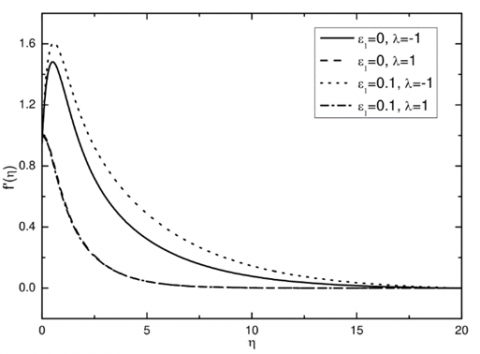(a)

## 1b.png(b)

## c.png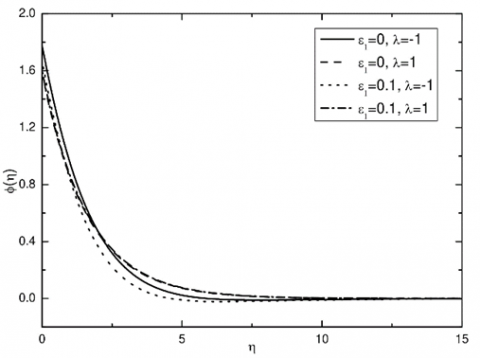(c)

Figure 1. Effect of thermal stratification on velocity, temperature and concentration profiles when Pr=0.72, Sc=0.22, Gr=5.0, Gc=5.0, ε=0.9, Da=0.1, A=45°, ε2=0.1

In Figure 3, the influence of angle made by stretching sheet on these profiles is shown. The effect of these parameters on non-dimensional physical quantities as Nusselt and Sherwood numbers with skin friction coefficients are shown in tabular form labelled as Table 1. The detailed discussion about each effect is given as follows:

## a.png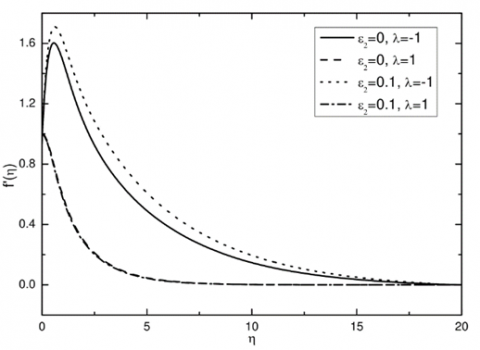(a)

## b.png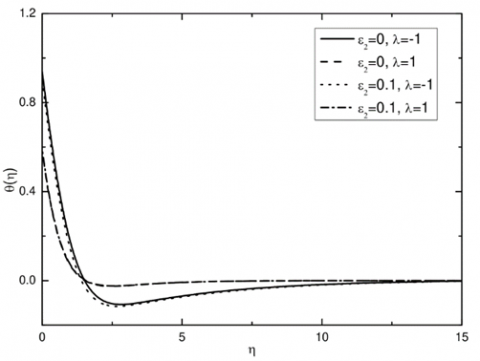(b)

## 2c.png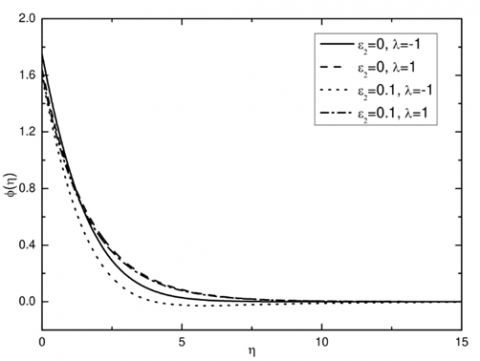(c)

Figure 2. Effect of solutal stratification on velocity, temperature and concentration profiles when Pr=0.72, Sc=0.22, Gr=5.0, Gc=5.0, ε=0.9, Da=0.1, A=45°, ε1=0.2

## 3a.png(a)

## 3b.png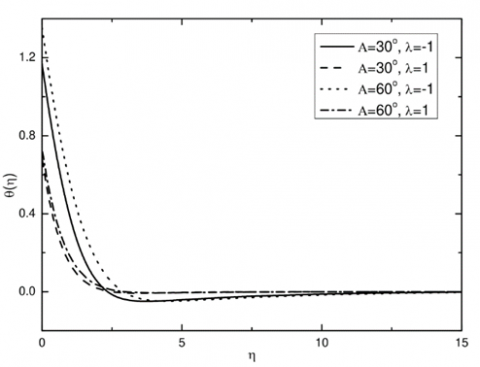(b)

## 3c.png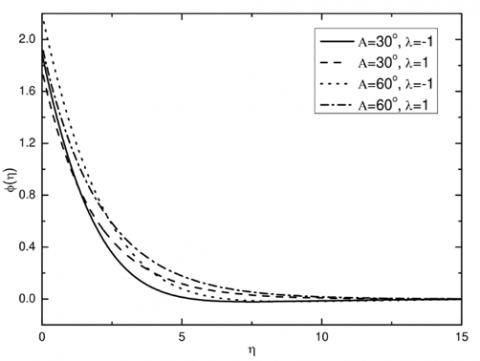(c)

Figure 3. Effects of change in acute angle A on velocity, temperature and concentration profiles when Pr=0.72, Sc=0.22, Gr=5.0, Gc=5.0, ε=0.9, Da=0.1, ε1=0.2, ε2=0.1

Table 1. Skin friction coefficient, Nusselt and Sherwood numbers for different combination of parameters

 ε1 ε2 A λ Cf Nux Shx 0 0.1 45° -1 0.8153 0.7238 0.4505 0.1 0.1 45° -1 1.0912 0.7499 0.4774 0 0.1 45° 1 -1.4879 1.3333 0.5202 0.1 0.1 45° 1 -1.5291 1.2425 0.5175 0.1 0 45° -1 0.8046 0.7188 0.4535 0.1 0.1 45° -1 1.0912 0.7499 0.4774 0.1 0 45° 1 -1.4938 1.2465 0.5366 0.1 0.1 45° 1 -1.5291 1.2425 0.5175 0.1 0.1 30° -1 1.6697 0.7881 0.5027 0.1 0.1 60° -1 0.2524 0.6871 0.4353 0.1 0.1 30° 1 -1.0988 1.2717 0.5366 0.1 0.1 60° 1 -2.1354 1.1966 0.4872

3.1 Effect of thermal stratification

The effect of thermal stratification on velocity, temperature and concentration profiles in respect of η are displayed in Figure 1. The λ=1 and -1 is taken to study this effect. All other parameters are fixed to physically relevant values as Pr=0.72, Sc=0.22, Gr=5.0, Gc=5.0, ε=0.9, Da=0.1, A=450, ε2=0.1. It is noted from the Figure 1(a) that for negative value of λ, there is sudden increase in the velocity which further reduces with increasing value of η in the absence and presence of thermal stratification. The velocity reaches to higher values in the presence of this parameter ε1 as compared to its absence. But there is no such thing in the case of positive λ. From Figure 1(b), it is visible that lower temperature is found in the presence of thermal stratification irrespective of positive or negative λ. Though, the maximum temperature obtained in the case of negative λ is far more than that of its positive value. In the physical sense, the presence of thermal stratification parameter makes the boundary layer thin because of emerged temperature difference. Figure 1(c) shows the concentration variation with respect to η values and it is observed that the presence of thermal stratification lowers the concentration and effect is more in the case of negative value of λ. All the physical quantities are increased in the presence of ε1 for negative λ and opposite nature is found of positive λ.

3.2 Effect of solutal stratification

The effect of solutal stratification on all the profiles of velocity, temperature and concentration in respect of η is displayed in Figure 2. The two values 1 and -1 are taken for λ in order to study this effect. Except the solutal stratification parameter ε2, all other involved parameters are fixed to certain values in their physical range as Pr=0.72, Sc=0.22, Gr=5.0, Gc=5.0, ε=0.9, Da=0.1, A=450, ε1=0.2. From Figure 2(a), it is clear that the impact of solutal stratification is almost similar to thermal stratification with enhanced peak value of velocity in its absence and presence for both negative and positive values of λ. The impact of ε2 on temperature and concentration profiles is also same as that of ε1 in all the cases with more negative values in a certain range of η. Like ε1 case, here also the physical quantities are increased in the presence of ε2 for negative λ and reverse is noticed for positive λ.

3.3 Effect of change in angle A

The velocity, temperature and concentration profiles for two acute angles of stretching sheet in the case of positive and negative λ, are shown in Figure 3. As in the previous cases, all other parameters are fixed to physically reliable values as Pr=0.72, Sc=0.22, Gr=5.0, Gc=5.0, ε=0.9, Da=0.1, ε1=0.2, ε2=0.1. It is observed from all these figures that for positive values of λ, profiles are almost similar for both the angles but in the case of negative λ, velocity profiles are much higher when the sheet is more horizontal. In the case of temperature and concentration profiles, these are decreased when the sheet approaches vertical direction. All the three physical quantities studied faced decrement for higher inclination angle A.

4. Conclusion

The important conclusion of this work can be pointed out as:

• When λ is negative i.e., in the case of injection velocity, the impact of solutal and thermal stratification are more in comparison with suction velocity (for positive λ). The similar behaviour is seen with temperature profiles but not with concentration profiles.
• In the angle variation, it is observed that the angle inclination of the stretching surface plays a very dominant role in the nature of profiles. When surface is more like horizontal i.e., A=30°, the velocity peak is much higher for injection case when compared to the case of surface being more like vertical i.e., A=60°. But the suction velocities are not much affected with angle variation of the sheet.
• The temperature is found to be more in the case of A=60° when compared to A=30° for both the injection and suction case.
• The similar behaviour is seen for the concentration profiles too for these two particular angles.
• The skin friction coefficient, Nusselt and Sherwood numbers are very much influenced with all these parameters and great change is found with angle variation A.
References

 Batchelor, C.K., Batchelor, G.K. (2000). An Introduction to Fluid Dynamics. Cambridge University Press.

 Ingham, D.B., Pop, I. (2005). Transport Phenomena in Porous Media III. Elsevier, Oxford.

 Nield, D.A., Bejan, A. (2017). Convection in Porous Media, Science and Technology, Springer.

 Crane, L.J. (1970). Flow past a stretching plate. Zeitschrift für Angewandte Mathematik und Physik ZAMP, 21(4): 645-647. https://doi.org/10.1007/BF01587695

 Pal, D., Hiremath, P.S. (2010). Computational modeling of heat transfer over an unsteady stretching surface embedded in a porous medium. Meccanica, 45(3): 415-424. https://doi.org/10.1007/s11012-009-9254-7

 RamReddy, C., Murthy, P.V.S.N., Rashad, A.M., Chamkha, A.J. (2016). Soret effect on stagnation-point flow past a stretching/shrinking sheet in a nanofluid-saturated non-Darcy porous medium. Special Topics & Reviews in Porous Media: An International Journal, 7(3): 229-243. https://doi.org/10.1615/SpecialTopicsRevPorousMedia.v7.i3.20

 Abo-Eldahab, E.M., El Aziz, M.A. (2004). Blowing/suction effect on hydromagnetic heat transfer by mixed convection from an inclined continuously stretching surface with internal heat generation/absorption. International Journal of Thermal Sciences, 43(7): 709-719. https://doi.org/10.1016/j.ijthermalsci.2004.01.005

 Ali, M., Alim, M.A., Alam, M.S. (2015). Similarity solution of heat and mass transfer flow over an inclined stretching sheet with viscous dissipation and constant heat flux in presence of magnetic field. Procedia Engineering, 105: 557-569. https://doi.org/10.1016/j.proeng.2015.05.089

 Rathish Kumar, B.V. (2005). Combined influence of mass and thermal stratification on double-diffusion non-Darcian natural convection from a wavy vertical wall to porous media. J. Heat Transfer, 127(6): 637-647. https://doi.org/10.1115/1.1863258

 Lakshmi Narayana, P.A., Murthy, P.V.S.N. (2006). Free convective heat and mass transfer in a doubly stratified non-Darcy porous medium. Journal of Heat Transfer, 128(11): 1204-1212. https://doi.org/10.1115/1.2352788

 Srinivasacharya, D., Surender, O. (2015). Double Stratification effects on mixed convection along a vertical plate in a non-Darcy porous medium. Procedia Engineering, 127: 986-993. https://doi.org/10.1016/j.proeng.2015.11.447

 Hayat, T., Qayyum, S., Imtiaz, M., Alsaedi, A. (2018). Double stratification in flow by curved stretching sheet with thermal radiation and joule heating. Journal of Thermal Science and Engineering Applications, 10(2): 021010. https://doi.org/10.1115/1.4037774

 Gray, D.D., Giorgini, A. (1976). The validity of the Boussinesq approximation for liquids and gases. International Journal of Heat and Mass Transfer, 19(5): 545-551. https://doi.org/10.1016/0017-9310(76)90168-X

 Motsa, S.S. (2013). A new spectral local linearization method for nonlinear boundary layer flow problems. Journal of Applied Mathematics, 2013: Article ID 423628. https://doi.org/10.1155/2013/423628

 Chetteti, R., Srivastav, A. (2021). Effect of dispersion on thermally stable stratified power-law fluids over the vertical frustum of a cone in a non-Darcy porous medium: Flow separation. Heat Transfer, 50(3): 2380-2402. https://doi.org/10.1002/htj.21983

 Zhou, B., Meng, E., Chen, Z. (2018). Numerical study on heat transfer performance of composite phase change energy storage capsule material. Fresenius Environmental Bulletion, 27(4): 2373-2379.